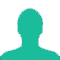A and B are two fixed points 5 cm apart and C is a point on AB such that AC is 3cm. if the length of AC is increased by 6%, the length of CB is decreased by

A. 6%

B. 7%

C. 8%

D. 9%

Solution(By Examveda Team)

As A and B are fixed, C is any point on AB, so if AC is increases then CB decreases.
A________3 cm_________ C _____2 cm____B
Then, solution can be visualized as,
Increase in AC 6% = $$\frac{{106 \times 3}}{{100}} = 3.18\,{\text{cm}}{\text{.}}$$
Decrease in CB = 0.18 cm
% decrease = $$\frac{{0.18}}{2} \times 100 = 9\%$$

Alternatively,
AC = 3 Cm.
BC = 2 Cm.
Increase in AC by 6%, then
New, AC = 3 + 6% of 3 = 3 + 0.18 = 3.18 cm.
0.18 cm increase in AC means 0.18 cm decrease in BC as already mentioned AB as the fixed point.
So, % decrease in BC,
\eqalign{ & = \frac{{{\text{Actual}}\,{\text{Decrease}}\,{\text{in}}\,{\text{BC}}}}{{{\text{Original BC}}}} \times 100 \cr & = \frac{{0.18}}{2} \times 100 = 9\% \cr}

1.Ab = 5 CM.
AC = 3 Cm.
BC = 2 CM.
6% increase in AC = 3 + 6% of 3 = 3.18 CM.
Increase in AC = 0.18 CM
So, decrease in BC = 0.18 as AB is fixed.
% decrease in BC = (0.18 *100)/2 = 9%. :)

2.decr.CB= 0.18 cm explan it and % decrease=0.18/2*100=9% there is what is 2

Related Questions on Percentage